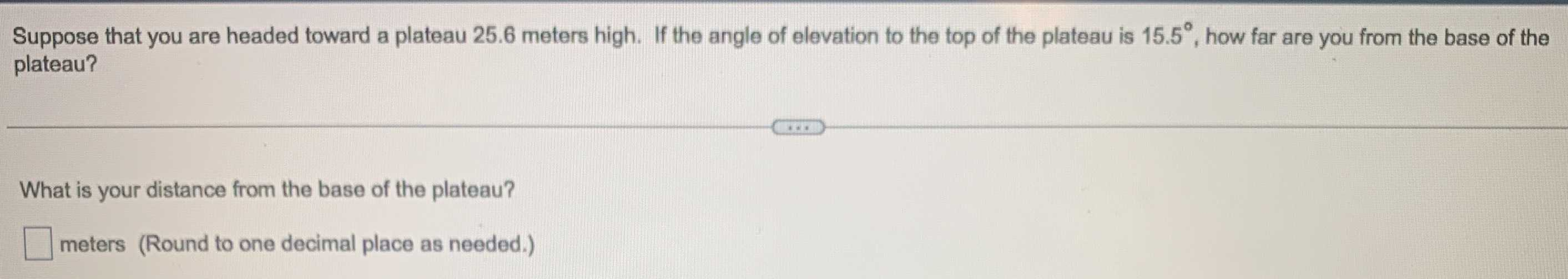### Still have math questions?

Trigonometry
QuestionSuppose that you are headed toward a plateau $$25.6$$ meters high. If the angle of elevation to the top of the plateau is $$15.5 ^ { \circ }$$ , how far are you from the base of the plateau?

What is your distance from the base of the plateau?

_____meters

(Round to one decimal place as needed.)